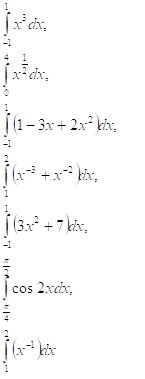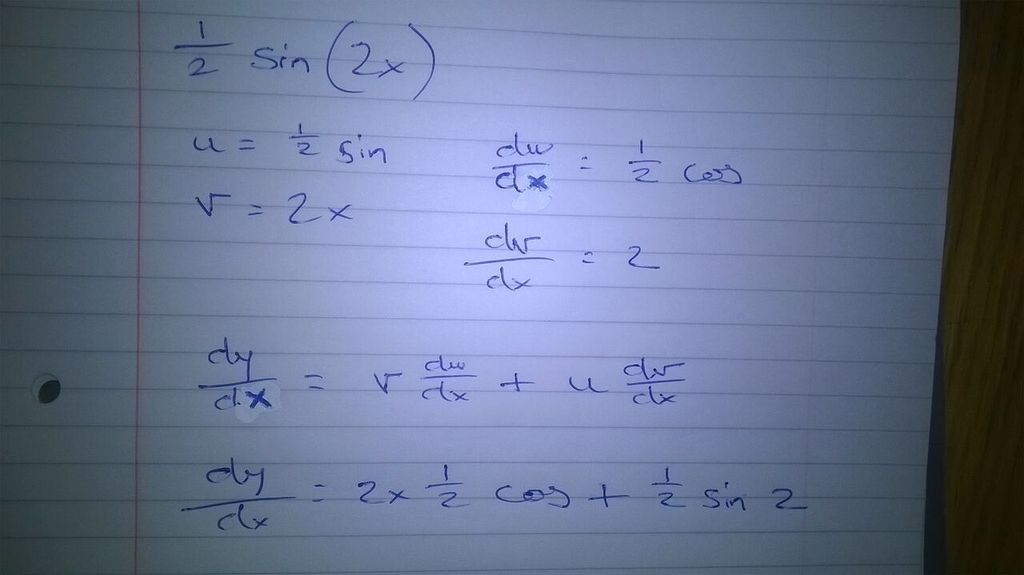User Name Remember Me? Password

 Calculus Calculus Math Forum

 October 17th, 2017, 10:32 AM #1 Member   Joined: Nov 2016 From: Ireland Posts: 84 Thanks: 3 Definite integrals Hi guys I'm working on these integrals.See this one second from the bottom, with the cos 2x dx? My lecturer has given the answer of $\displaystyle [1/2 \sin 2x]$ I'm thinking this should be $\displaystyle [2/2 \sin 2x]$Last edited by skipjack; October 17th, 2017 at 01:00 PM.October 17th, 2017, 10:36 AM #2 Senior Member   Joined: Sep 2015 From: USA Posts: 2,531 Thanks: 1390 Your lecturer is correct. Thanks from KevineamonOctober 17th, 2017, 11:05 AM #3 Member   Joined: Nov 2016 From: Ireland Posts: 84 Thanks: 3 Lol thx Rom - Ok say it was the 2x on it's own = $\displaystyle 2x^1$ Integral $\displaystyle 2x^1$ = $\displaystyle (2x^2)/2$ Right? So what am I missing here? how does the trigonometric function change that?October 17th, 2017, 11:36 AM   #4
Senior Member

Joined: Sep 2015
From: USA

Posts: 2,531
Thanks: 1390

Quote:
 Originally Posted by KevineamonLol thx Rom - Ok say it was the 2x on it's own = $\displaystyle 2x^1$ Integral $\displaystyle 2x^1$ = $\displaystyle (2x^2)/2$ Right? So what am I missing here? how does the trigonometric function change that?
oy...

you can't apply polynomial derivative rules to trig functions.

Just take the derivative of $\sin(2x)$ vs. $\dfrac 1 2 \sin(2x)$ and see which one gets you $\cos(2x)$October 17th, 2017, 12:32 PM #5 Member   Joined: Nov 2016 From: Ireland Posts: 84 Thanks: 3 Hmmm I'm a bit of a n00bee with these Rom According to my calculations this is the derivative of: sin(2x)October 17th, 2017, 12:55 PM #6 Math Team   Joined: Dec 2013 From: Colombia Posts: 7,675 Thanks: 2655 Math Focus: Mainly analysis and algebra You know that $\sin(2x)$ is the sine of $2x$? It's not $\sin{}$ times $2x$. $\sin{}$ on its own is meaningless. It needs an argument. You'd use the chain rule for that derivative. Thanks from topsquark Last edited by v8archie; October 17th, 2017 at 12:58 PM.October 17th, 2017, 01:34 PM #7 Member   Joined: Nov 2016 From: Ireland Posts: 84 Thanks: 3 Hmm k - I wonder if someone could give me the answers to Roms questions, in a short step format. I seem to have unlearned everything I thought I learned. Sorry guysOctober 17th, 2017, 01:53 PM #8 Senior Member   Joined: Sep 2015 From: USA Posts: 2,531 Thanks: 1390 $\dfrac {d}{dx}\left( \sin(2x)\right) = \cos(2x)\cdot (2) = 2 \cos(2x)$ $\dfrac {d}{dx}\left( \dfrac 1 2 \sin(2x)\right) = \dfrac 1 2 \cos(2x) \cdot 2 = \cos(2x)$ soooo $\displaystyle \int \cos(2x)~dx = \dfrac 1 2 \sin(2x)$October 17th, 2017, 03:40 PM #9 Math Team   Joined: Jan 2015 From: Alabama Posts: 3,264 Thanks: 902 If your lecture actually said that the "answer" to this problem is $\displaystyle (1/2)\sin(2x)$ that is incorrect! This is, as you say in the title of this thread, a definite integral so the "answer" is a number, not a function of x! To integrate $\displaystyle \int_{\pi/4}^{\pi/2} \cos(2x) dx$, let $\displaystyle u= 2x$. Then $\displaystyle du= 2dx$ so $\displaystyle dx= \frac{1}{2}du$. When $\displaystyle x= \pi/4$, $\displaystyle u= 2(\pi/4)= \pi/2$ and when $\displaystyle x= \pi/2$ $\displaystyle u= 2(\pi/2)= \pi$. The integral becomes $\displaystyle \frac{1}{2}\int_{\pi/2}^{\pi} \cos(u)du= \left[(1/2) \sin(u)\right]_{\pi/2}^{\pi}= \frac{1}{2}(\sin(\pi)- \sin(\pi/2))= \frac{1}{2}(0- 1)= -\frac{1}{2}$. If you really, as v8archie suggested, were trying to do this as "sin" times "2x", that is very troubling. You should have immediately recognized that "sin" without any "x" is not a function at all! It is just three meaningless letters! Last edited by skipjack; October 18th, 2017 at 11:29 AM.Tags definite, integralsThread ToolsShow Printable VersionEmail this Page Display ModesLinear ModeSwitch to Hybrid ModeSwitch to Threaded ModeSimilar Threads Thread Thread Starter Forum Replies Last Post Agata78 Calculus 6 January 19th, 2013 02:05 PM Agata78 Calculus 18 January 18th, 2013 12:39 PM jakeward123 Calculus 10 February 28th, 2011 12:18 PM Aurica Calculus 2 May 10th, 2009 05:05 PM Agata78 Abstract Algebra 0 December 31st, 1969 04:00 PM

 Contact - Home - Forums - Cryptocurrency Forum - Top

Copyright © 2019 My Math Forum. All rights reserved.